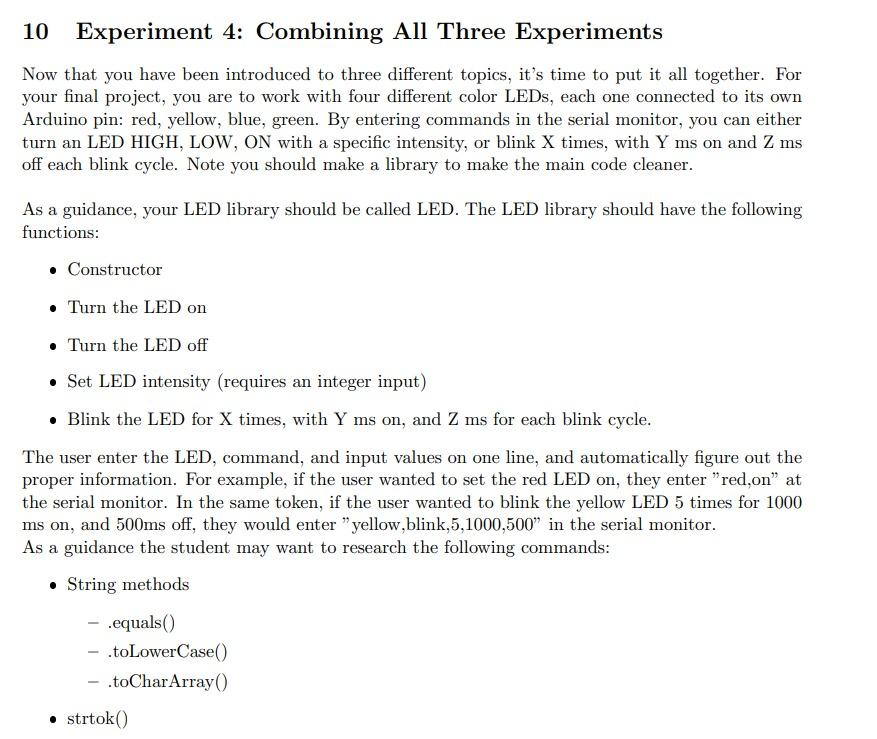# Question Solved1 Answer10 Experiment 4: Combining All Three Experiments Now that you have been introduced to three different topics, it's time to put it all together. For your final project, you are to work with four different color LEDs, each one connected to its own Arduino pin: red, yellow, blue, green. By entering commands in the serial monitor, you can either turn an LED 10 Experiment 4: Combining All Three Experiments Now that you have been introduced to three different topics, it's time to put it all together. For your final project, you are to work with four different color LEDs, each one connected to its own Arduino pin: red, yellow, blue, green. By entering commands in the serial monitor, you can either turn an LED HIGH, LOW, ON with a specific intensity, or blink X times, with $$\mathrm{Y} \mathrm{ms}$$ on and $$\mathrm{Z} \mathrm{ms}$$ off each blink cycle. Note you should make a library to make the main code cleaner. As a guidance, your LED library should be called LED. The LED library should have the following functions: - Constructor - Turn the LED on - Turn the LED off - Set LED intensity (requires an integer input) - Blink the LED for $$\mathrm{X}$$ times, with $$\mathrm{Y} \mathrm{ms}$$ on, and $$\mathrm{Z}$$ ms for each blink cycle. The user enter the LED, command, and input values on one line, and automatically figure out the proper information. For example, if the user wanted to set the red LED on, they enter "red,on" at the serial monitor. In the same token, if the user wanted to blink the yellow LED 5 times for 1000 $$\mathrm{ms}$$ on, and $$500 \mathrm{~ms}$$ off, they would enter "yellow,blink, $$5,1000,500$$ " in the serial monitor. As a guidance the student may want to research the following commands: - String methods $$-$$. equals () - .toLowerCase() - .toCharArray()ECPIMI The Asker · Computer Science​​​​​​​By using Arduino

Transcribed Image Text: 10 Experiment 4: Combining All Three Experiments Now that you have been introduced to three different topics, it's time to put it all together. For your final project, you are to work with four different color LEDs, each one connected to its own Arduino pin: red, yellow, blue, green. By entering commands in the serial monitor, you can either turn an LED HIGH, LOW, ON with a specific intensity, or blink X times, with $$\mathrm{Y} \mathrm{ms}$$ on and $$\mathrm{Z} \mathrm{ms}$$ off each blink cycle. Note you should make a library to make the main code cleaner. As a guidance, your LED library should be called LED. The LED library should have the following functions: - Constructor - Turn the LED on - Turn the LED off - Set LED intensity (requires an integer input) - Blink the LED for $$\mathrm{X}$$ times, with $$\mathrm{Y} \mathrm{ms}$$ on, and $$\mathrm{Z}$$ ms for each blink cycle. The user enter the LED, command, and input values on one line, and automatically figure out the proper information. For example, if the user wanted to set the red LED on, they enter "red,on" at the serial monitor. In the same token, if the user wanted to blink the yellow LED 5 times for 1000 $$\mathrm{ms}$$ on, and $$500 \mathrm{~ms}$$ off, they would enter "yellow,blink, $$5,1000,500$$ " in the serial monitor. As a guidance the student may want to research the following commands: - String methods $$-$$. equals () - .toLowerCase() - .toCharArray()
More
Transcribed Image Text: 10 Experiment 4: Combining All Three Experiments Now that you have been introduced to three different topics, it's time to put it all together. For your final project, you are to work with four different color LEDs, each one connected to its own Arduino pin: red, yellow, blue, green. By entering commands in the serial monitor, you can either turn an LED HIGH, LOW, ON with a specific intensity, or blink X times, with $$\mathrm{Y} \mathrm{ms}$$ on and $$\mathrm{Z} \mathrm{ms}$$ off each blink cycle. Note you should make a library to make the main code cleaner. As a guidance, your LED library should be called LED. The LED library should have the following functions: - Constructor - Turn the LED on - Turn the LED off - Set LED intensity (requires an integer input) - Blink the LED for $$\mathrm{X}$$ times, with $$\mathrm{Y} \mathrm{ms}$$ on, and $$\mathrm{Z}$$ ms for each blink cycle. The user enter the LED, command, and input values on one line, and automatically figure out the proper information. For example, if the user wanted to set the red LED on, they enter "red,on" at the serial monitor. In the same token, if the user wanted to blink the yellow LED 5 times for 1000 $$\mathrm{ms}$$ on, and $$500 \mathrm{~ms}$$ off, they would enter "yellow,blink, $$5,1000,500$$ " in the serial monitor. As a guidance the student may want to research the following commands: - String methods $$-$$. equals () - .toLowerCase() - .toCharArray()